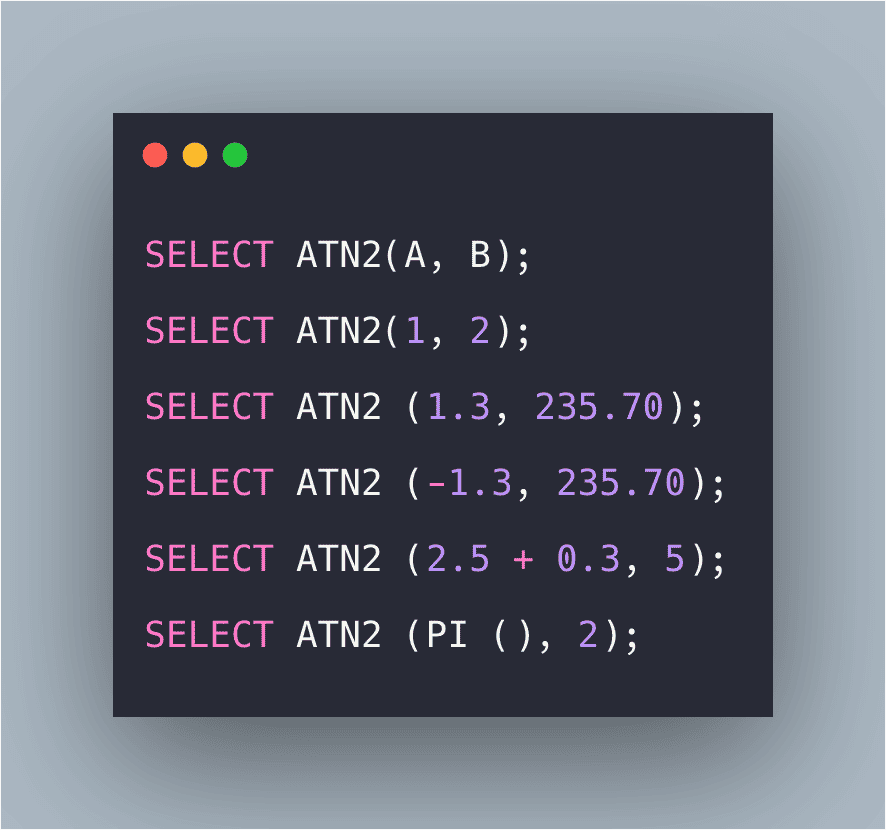# SQL ATN2() Function: The Complete Guide

0
2The SQL ATN2 function is a SQL Mathematical Function which returns the angle between the positive x-axis and the line from the origin to the point (y, x).

## SQL ATN2()

SQL ATN2() is a built-in function that is used for finding the arctangent of two numbers i.e. it returns the angle, in radians, between the positive x-axis and the ray from the origin to the point (y, x), where x and y are the values or points of the two specified float expressions.

SQL ATN2() function returns the arctangent of two numbers.

### Syntax

```SELECT ATN2(A, B);
```

### Parameters

A, B: Two values whose arctangent value is to be calculated.

### Examples

#### Query 1

```SELECT ATN2(1, 2);
```

#### Output

```0.46364760900080609
```

#### Explanation

Here, the arc tangent of both the values is returned.

#### Query 2

```SELECT ATN2 (1.3, 235.70);
```

#### Output

```0.00551542985993961
```

#### Explanation

Here, arc tangent of both the values is returned.

#### Query 3

```SELECT ATN2 (-1.3, 235.70);
```

#### Output

```-5.5154298599396114E-3
```

#### Explanation

Here, arctangent value is returned which is negative.

#### Query 4

```SELECT ATN2 (2.5 + 0.3, 5);
```

#### Output

```0.510488321916776
```

#### Explanation

Here, the arc tangent value of the regular expression is returned.

#### Query 5

```SELECT ATN2 (PI (), 2);
```

#### Output

```1.00388482185389
```

#### Explanation

Here, the arc tangent value of the function PI () is returned with another normal expression.